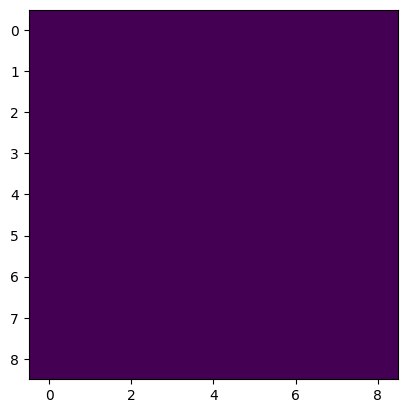# The output of Tensor.mean() are different according to the position

When I was checking the feature maps of my model, I found something bizarre:

Although each channel of the input tensor X (the size of x is 256 * 9 * 9, C * H * W) is a constant value. The result of X.mean(0) is not in accord with expectations. The following is the result of plt.imshow(X.mean(0).numpy()):What this visualized result tells us is that the value in X.mean(0) are different according to the position although their inputs are the same. Actually the value of X.mean(0)[0, 0] is 0.07183486223220825, while the value of X.mean(0)[-1, -1] is 0.07183484733104706 (their difference is 1.4901161e-08).

I know this difference is very very tiny, but I am curious why there is a difference.
The torch version is 1.8.2+cu102 and the numpy version is 1.20.3.

In addition, the result of plt.imshow(X.numpy().mean(0)) is as follows:Here is a binary file in NumPy `.npy` format of the input X. You can use X = np.load(filepath) to load it and X = torch.FloatTensor(X) to transform X from ndarray to tensor.

Each channel of the input tensor X is a constant value like this:

And I found that when H and W of the input tensor are 2 to the power of N (N is nonnegative integer), the result of X.mean(0) will be correct.

The small absolute errors are most likely created by the limited floating point precision and due to a different order of operations.

Thanks for your reply. But there should be no precision loss between torch.float32 and numpy.float32. And the result of

``````import numpy as np
import torch

plt.imshow(torch.FloatTensor(X).numpy().mean(0))
plt.show()
``````

is as follows:So I think there is no precision loss whether it is from torch.float32 to numpy.float32 or vice versa. I think the real problem is the difference between the algorithm of torch.mean() and numpy.mean().

Yes, there won’t be a precision loss while transforming between numpy as PyTorch, since `from_numpy` and `tensor.numpy()` would share the underlying data. The difference would come from potentially different implementations (and order of operations) in the `mean` op.

So how could I know the implementation of torch.mean()?

I think it’s definition can be found here.

Many thanks for your detailed answer!